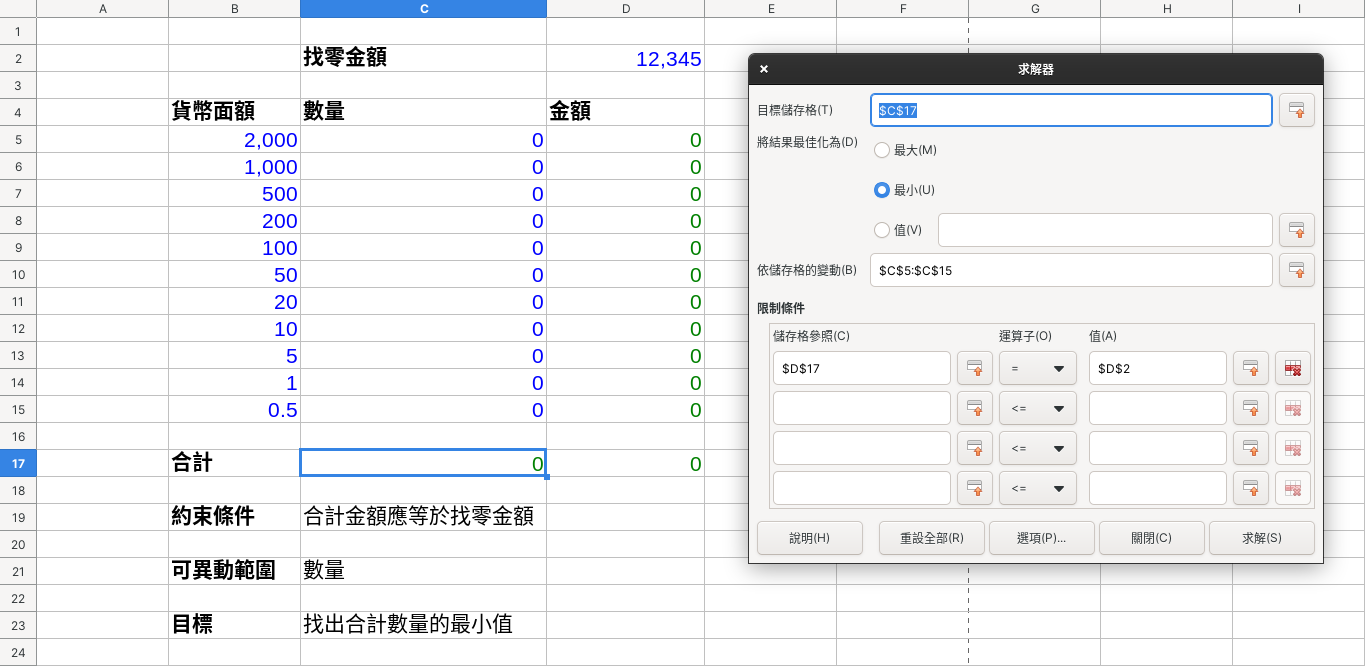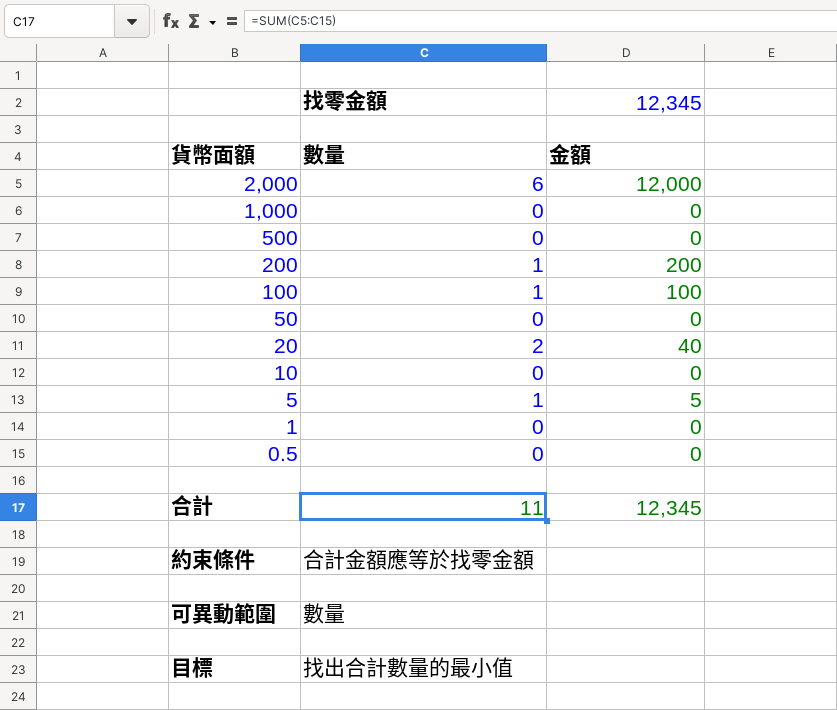## 規劃問題與求解器• 排班、排程、排工問題，怎麼排才能公平又滿足多方需求？
• 路徑規劃問題，怎麼走才能讓運輸效率最大化？
• 裝箱問題，怎麼裝才能讓箱體利用率最大化？
• 裁切問題，怎麼切才能讓物料利用率最大化？
• 賽事問題，怎麼排才能讓賽事公平而不強碰？
• 投資問題，怎麼配置才能讓收益最大化？

## OR-Tools

OR-Tools 是 Google 開發的求解器，名字中的「OR」表示 operations research（作業研究），這是一門科學化探討各種作業問題的學科。OR-Tools 本身是以 C++ 開發，另提供多種語言的 API，由於 C++ 可直接編譯成各平台的原生二進位檔，因此不像 OptaPy 那樣還要等 JVM 跑起來，OR-Tools 即使在世界知名的慢速語言 Python 下跑起來都可以秒解問題，既提高了效率，也減少了客怨。

OR-Tools 內集合了多個求解器，每個求解器就是一套演算法，它們各有擅長之處，有的適合解線性問題，有的適合解約束問題，因為本人的需求為約束問題，所以下面只會談到 OR-Tools 中的約束問題求解器 CP-SAT。

``````\$ pip install ortools
``````

## OR-Tools 的 CP-SAT

CP-SAT 是 OR-Tools 內建的求解器之一，「CP」表示 constraint programming（約束編程）、「SAT」表示 Boolean satisfiability problem（布林可滿足性問題），這些專業名詞有點艱澀，簡單說就是上面舉的那些規劃問題，而 CP-SAT 就是針對這類問題的求解器。

1. 創建變數
2. 制定約束
3. 制定目標
4. 執行求解

## 簡單約束問題

• 有 x、y、z 三個變數，它們的值可以是 0、1、2。
• x 不等於 y。
• x + y + z 最大可能是多少？

``````"""Simple solve"""
from ortools.sat.python import cp_model

"""Minimal CP-SAT example to showcase calling the solver"""
# Create solver model
model = cp_model.CpModel()
``````

``````# Create the variable.
x = model.NewIntVar(lb=0, ub=2, name='x')
y = model.NewIntVar(lb=0, ub=2, name='y')
z = model.NewIntVar(lb=0, ub=2, name='z')
``````

``````# Create the constraints
``````

``````# Objective
model.Maximize( x + y + z )
``````

``````# Create a solver and solve the model
solver = cp_model.CpSolver()
status = solver.Solve(model=model)
``````

``````if status == cp_model.OPTIMAL or status == cp_model.FEASIBLE:
print(f'Maximum of objective function: {solver.ObjectiveValue()}\n')
print(f'x = {solver.Value(expression=x)}')
print(f'y = {solver.Value(expression=y)}')
print(f'z = {solver.Value(expression=z)}')
else: print('No solution found.')
``````

``````Maximum of objective function: 5.0

x = 1
y = 2
z = 2
``````

``````# Statistics
print(solver.ResponseStats())
``````

``````CpSolverResponse summary:
status: OPTIMAL
objective: 5
best_bound: 5
integers: 1
booleans: 0
conflicts: 0
branches: 0
propagations: 0
integer_propagations: 1
restarts: 0
lp_iterations: 0
walltime: 0.00235364
usertime: 0.00235602
deterministic_time: 0
gap_integral: 0
solution_fingerprint: 0xbc76f3d3e780d9b7
``````

``````"""Simple solve"""
from ortools.sat.python import cp_model

"""Minimal CP-SAT example to showcase calling the solver"""
# Create solver model
model = cp_model.CpModel()

# Create the variable.
x = model.NewIntVar(lb=0, ub=2, name='x')
y = model.NewIntVar(lb=0, ub=2, name='y')
z = model.NewIntVar(lb=0, ub=2, name='z')

# Create the constraints

# Objective
model.Maximize( x + y + z )

# Create a solver and solve the model
solver = cp_model.CpSolver()
status = solver.Solve(model=model)

if status == cp_model.OPTIMAL or status == cp_model.FEASIBLE:
print(f'Maximum of objective function: {solver.ObjectiveValue()}\n')
print(f'x = {solver.Value(expression=x)}')
print(f'y = {solver.Value(expression=y)}')
print(f'z = {solver.Value(expression=z)}')
else: print('No solution found.')

# Statistics
print(solver.ResponseStats())
``````